• OpenCV调整图像对比度和亮度一、学习目标二、原理理解三、对比度亮度调整四、完整代码示例 一、学习目标 理解图像对比度和亮度调整的原理 对比三种不同亮度和对比度调整方法 二、原理理解 1、对比度 对比度指的...


OpenCV调整图像对比度和亮度
一、学习目标二、原理理解三、对比度、亮度调整四、完整代码示例

一、学习目标
理解图像对比度和亮度调整的原理对比三种不同亮度和对比度调整方法
二、原理理解
1、对比度 对比度指的是一幅图像中明暗区域最亮的白和最暗的黑之间不同亮度层级的测量，差异范围越大代表对比越大，差异范围越小代表对比越小，好的对比率120:1就可容易地显示生动、丰富的色彩，当对比率高达300:1时，便可支持各阶的颜色。但对比率遭受和亮度相同的困境，现今尚无一套有效又公正的标准来衡量对比率，所以最好的辨识方式还是依靠使用者眼睛。
2、亮度 图象亮度是指画面的明亮程度，单位是堪德拉每平米(cd/m2)或称nits。图象亮度是从白色表面到黑色表面的感觉连续体，由反射系数决定，亮度侧重物体，重在“反射”。亮度是一副图像给人的一种直观感受，如果是灰度图像，则跟灰度值有关，灰度值越高则图像越亮。在RGB图像中，亮度体现为每个点的像素值的大小，像素值越大，亮度越高。
3、对比度和亮度调整公式参数α>0和β通常被称为增益参数和偏置参数；有时这些参数被称为分别控制对比度和亮度。 可以认为 f(x) 是源图像像素，g(x) 是输出图像像素。然后，我们可以更方便地将表达式写成：其中i和j表示像素位于第i行和第j列。α 作为系数乘以源像素值，会扩大最大像素值和最小像素值之间的差异，从而提升对比度。β 作为加数，直接增大源像素值，能提升亮度。
三、对比度、亮度调整
1、直接套用公式实现
for( int y = 0; y < image.rows; y++ ) {
for( int x = 0; x < image.cols; x++ ) {
for( int c = 0; c < image.channels(); c++ ) {
new_image.at<Vec3b>(y,x)[c] =
saturate_cast<uchar>( alpha*image.at<Vec3b>(y,x)[c] + beta );
}
}
}

执行操作 g(i,j) = α ⋅ f(i,j) + β ，访问图像中的每个像素。因为我们是在操作BGR图像，所以每个像素有三个值(B, G和R)，所以我们需要分别访问它们。
2、使用 cv::Mat::convertTo() 函数 cv::Mat::convertTo 的函数原型为：
void cv::Mat::convertTo	(OutputArray 	m,
int 			rtype,
double 		alpha = 1,
double 		beta = 0)	const

参数 m：输出矩阵；如果在操作之前没有正确的大小或类型，则重新分配。参数 rtype：所需的输出矩阵类型，或者更确切地说，深度，通道的数量与输入相同;如果rtype为负，则输出矩阵将与输入矩阵具有相同的类型。参数 alpha：可选的比例因子，用于调整对比度。参数 beta：可选的缩放因子，用于调整亮度。 该函数是Mat对象的一个方法，用于将Mat的数组转换为另一种可选伸缩的数据类型。 该方法将源像素值转换为目标数据类型。其转换公式为:satate_cast <> 应用于最后以避免可能的数值溢出。计算公式基本与对比度和亮度调整公式一致。
调用代码如下：
image.convertTo(new_image, -1, alpha, beta);

3、现有公式的问题分析及改进
公式理解
增加(/减少) β 值将增加(/减少)一个常量值到每个像素。超过 [0，255] 范围的像素将过于饱和(即大于(或小于)255(/ 0)的像素值将被调整为255(/ 0))。浅灰色为原始图像的像素值分布直方图，Gimp中亮度= 80时为深灰色

直方图表示每种颜色级别的像素数量。一个黑暗的图像将有许多低颜色值的像素，因此直方图将在其左侧呈现一个峰值。当添加一个恒定的偏置 β 时，直方图会向右移动，因为我们已经向所有像素添加了恒定的偏置。
α 参数将改变直方图的水平扩展。如果α<1，颜色级别将被压缩，结果将是一个对比度较低的图像。浅灰色为原始图像的直方图，Gimp中对比度< 0时为深灰色

你会发现，调整β 偏置值将提高亮度，但同时图像会感觉蒙上了一层纱，因为对比度降低，α增益可以用来减小这种影响，但由于饱和，我们会丢失一些原始亮区的细节。
γ 校正
Gamma校正可以使用输入值和映射输出值之间的非线性转换来校正图像的亮度：由于这种关系是非线性的，所有像素的效果将不相同，并将取决于它们的原始值。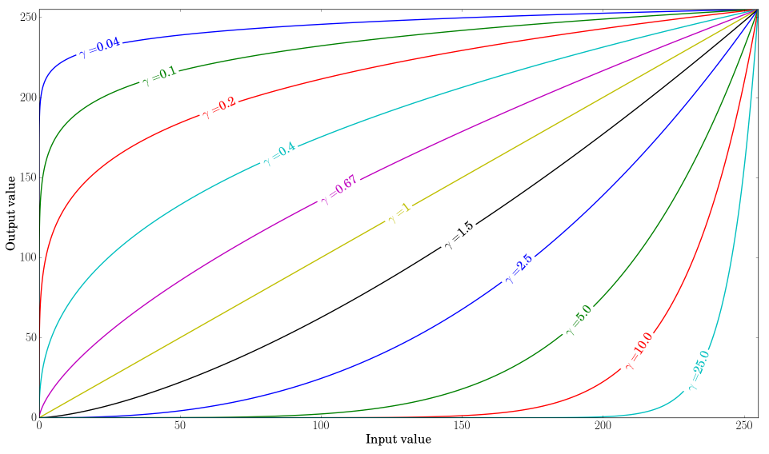绘制不同的伽玛值

当 γ<1 时，原始的暗区会变亮，直方图会向右移动，而γ>1 与之相反。
使用查找表实现 γ校正
 Mat lookUpTable(1, 256, CV_8U);
uchar* p = lookUpTable.ptr();
for( int i = 0; i < 256; ++i)
p[i] = saturate_cast<uchar>(pow(i / 255.0, gamma_) * 255.0);
Mat res = img.clone();
LUT(img, lookUpTable, res);

效果对比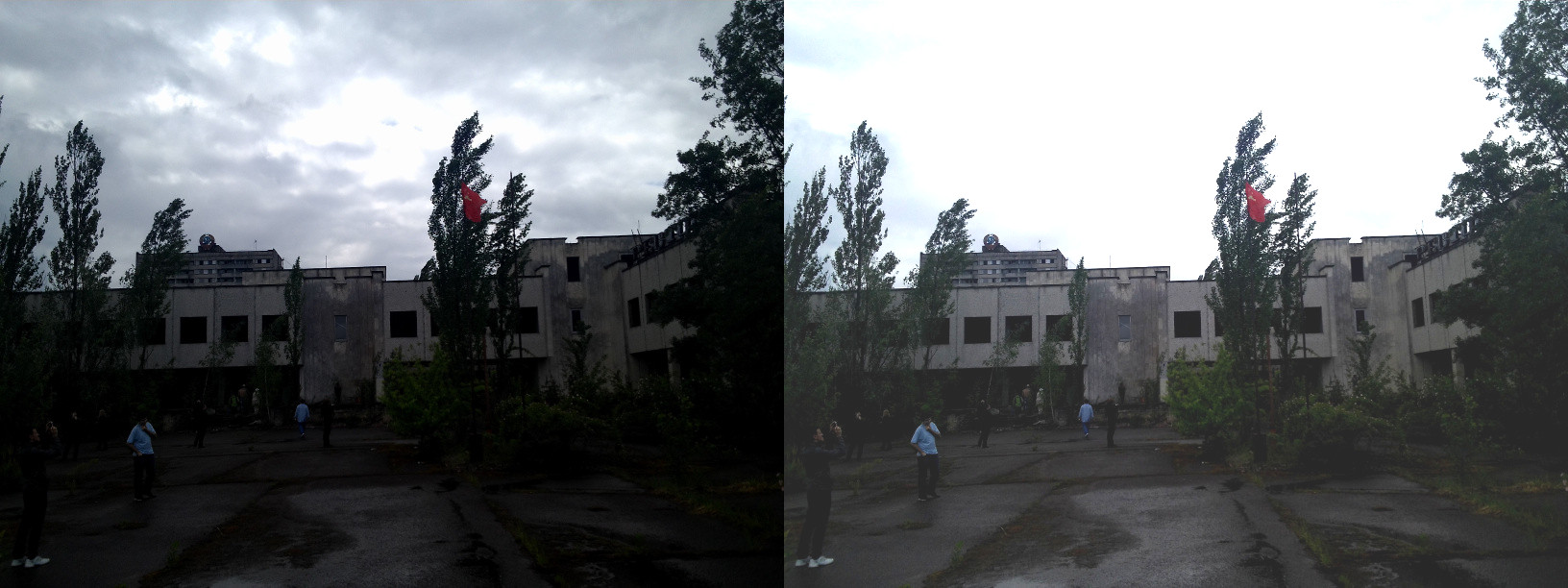上图是直接套用公式调整对比度和亮度的效果，其中α=1.3 ，β=40。整体的亮度得到了提高，但是你可以注意到，由于存在数值饱和度（saturate_cast），云层已经非常饱和了。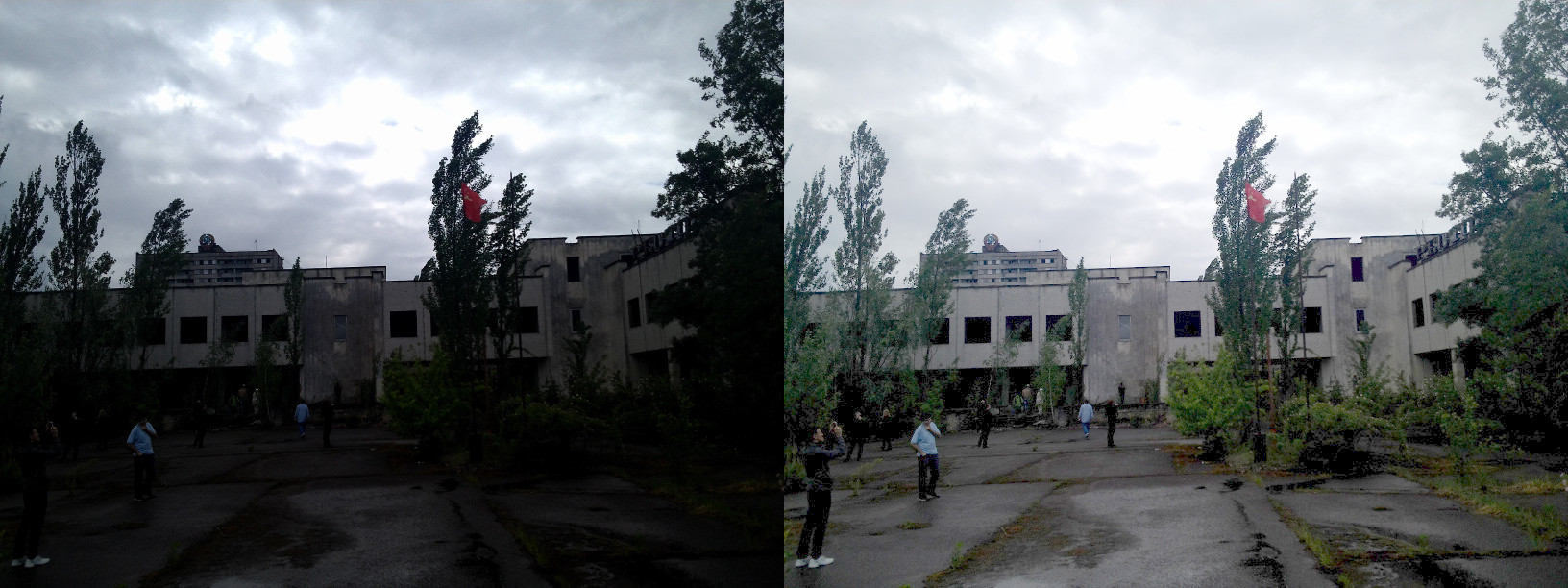上图是γ校正的效果，其中γ=0.4。gamma校正应该倾向于增加较少的饱和效应，因为映射是非线性的，而且不可能像在前一种方法中的数值饱和。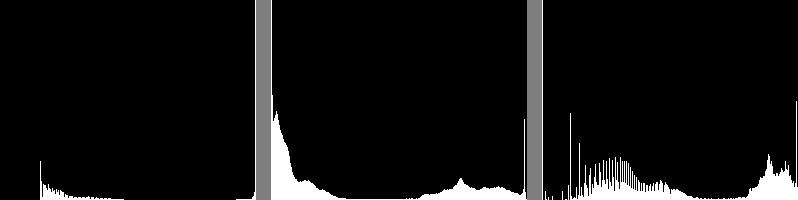左:alpha, beta修正后直方图;中心:原始图像的直方图;右:gamma校正后的直方图

上图比较了三幅图的直方图(其中y的取值范围不同)。您可以注意到，大多数像素值都位于原始图像直方图的下方。经过α，β校正后，由于饱和和右移，我们可以在255处观察到一个大的峰值。经过伽马校正后，直方图向右移动，但黑暗区域的像素比明亮区域的像素移动更大(见伽马曲线图)。
四、完整代码示例
#include<opencv2/opencv.hpp>
using namespace cv;
using namespace std;

int main(int argc, char** argv)
{
// 读入图像，判断读入是否成功
string fileName = samples::findFile("O:\\CSDN\\7.jpg");
if (src.empty())
{
fprintf(stderr, "failed to load image: %s\n", fileName);
system("pause");
return EXIT_FAILURE;
}

Mat dst1, dst2, dst3;
dst1 = Mat::zeros(src.size(), src.type());
double alpha = 1.0;
double beta = 0.0;
double gama = 1.0;

// 提示并输入 α  β  γ  的值
cout << " Basic Linear Transforms " << endl;
cout << "-------------------------" << endl;
cout << "* Enter the alpha value [1.0-3.0]: "; cin >> alpha;
cout << "* Enter the beta value [0-100]: ";    cin >> beta;
cout << "* Enter the gama value [-1,1]: ";    cin >> gama;

// 直接使用循环遍历每一个像素，应用公式
double t1 = (double)getTickCount();
for (int row=0;row<src.rows;++row)
for(int col=0;col<src.cols;++col)
for (int channel = 0; channel < src.channels(); ++channel)
{
dst1.at<Vec3b>(row, col)[channel] = saturate_cast<uchar>(alpha * src.at<Vec3b>(row, col)[channel] + beta);
}
double time1 = ((double)getTickCount() - t1) / getTickFrequency();
cout << "Method by pixel use time:" << time1 << "(ms)" << endl;

// 调用 convertTo() 函数调整对比度和亮度
double t2 = (double)getTickCount();
src.convertTo(dst2, -1, alpha, beta);
double time2 = ((double)getTickCount() - t2) / getTickFrequency();
cout << "Method by pixel use time:" << time2 << "(ms)" << endl;

// 构建查找表
Mat lookUpTable(1, 256, CV_8U);
uchar* p = lookUpTable.ptr();
for (int i = 0; i < 256; ++i)
p[i] = saturate_cast<uchar>(pow(i / 255.0, gama) * 255.0);

// 使用查找表进行对比度亮度调整
double t3 = (double)getTickCount();
LUT(src, lookUpTable, dst3);
double time3 = ((double)getTickCount() - t3) / getTickFrequency();
cout << "Method by γ correct use time:" << time3 << "(ms)" << endl;

// 调整窗体大小，显示调整效果
namedWindow("original", WINDOW_NORMAL);
resizeWindow("original", Size(src.cols / 2, src.rows / 2));
imshow("original", src);
namedWindow("pixel set", WINDOW_NORMAL);
resizeWindow("pixel set", Size(src.cols / 2, src.rows / 2));
imshow("pixel set", dst1);
namedWindow("convertTo", WINDOW_NORMAL);
resizeWindow("convertTo", Size(src.cols / 2, src.rows / 2));
imshow("convertTo", dst2);
namedWindow("γ correct", WINDOW_NORMAL);
resizeWindow("γ correct", Size(src.cols / 2, src.rows / 2));
imshow("γ correct", dst3);
waitKey(0);
system("pause");
return EXIT_SUCCESS;
}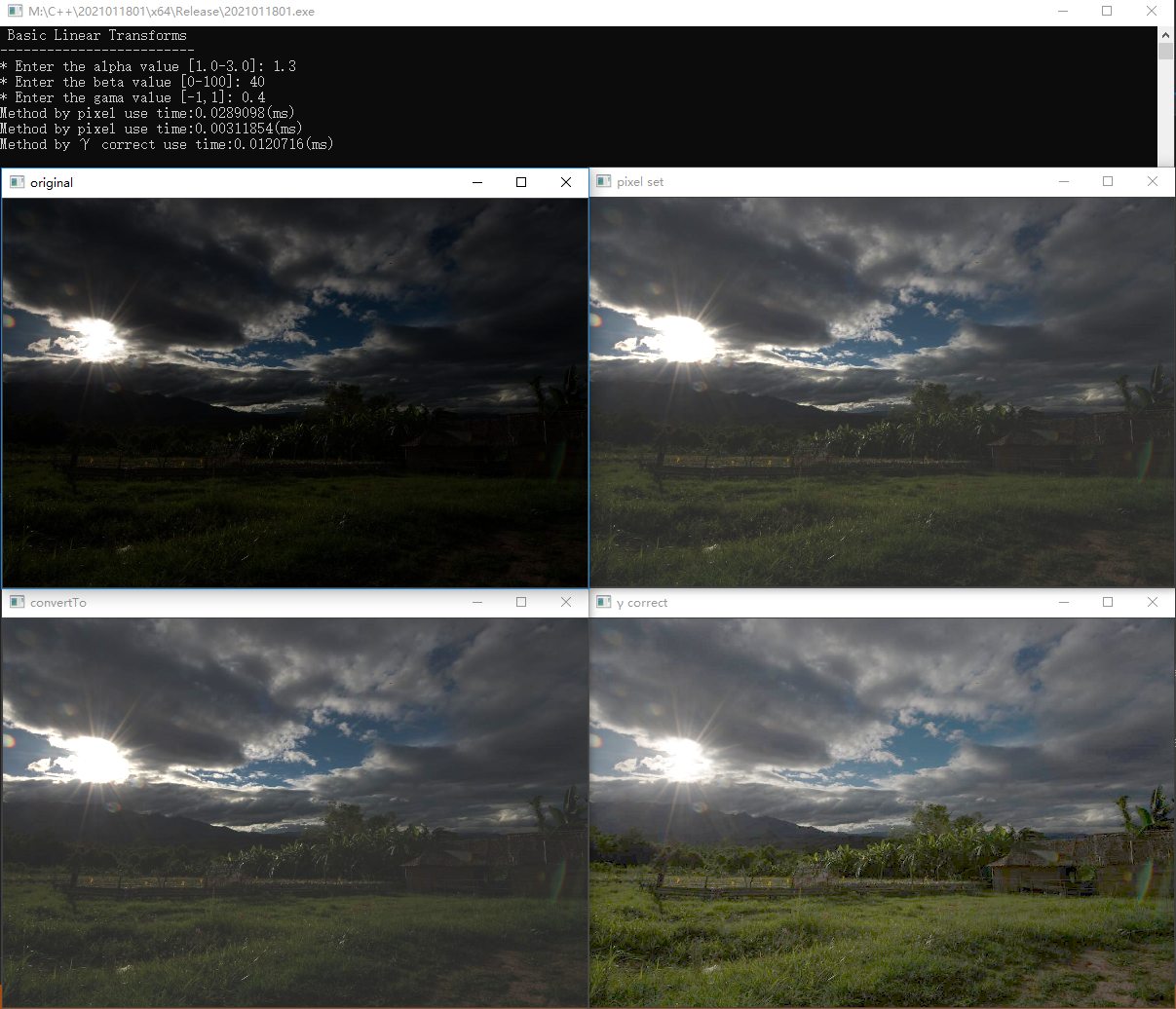展开全文OpenCV笔记 OpenCV教程
• 学习到范例五的时候，发觉虽然范例都很简单，但是做记录的时候，并且把程序里面使用过的类或方法都弄明白，也就不简单了，接下来介绍一下范例五吧。 1、图像处理 一般来说，图像处理算子是带有...2、亮度和对比度调整
学习到范例五的时候，发觉虽然范例都很简单，但是做记录的时候，并且把程序里面使用过的类或方法都弄明白，也就不简单了，接下来介绍一下范例五吧。
1、图像处理
一般来说，图像处理算子是带有一幅或多幅输入图像、产生一幅输出图像的函数。
图像变换可分为以下两种：
点算子（像素变换）：图像对比度和亮度，等等
邻域（基于区域的）算子：均值滤波，中值滤波，等等，也就是卷积运算
2、亮度和对比度调整
两种常用的点过程（即点算子），是用常数对点进行 乘法 和 加法 运算：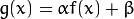两个参数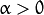和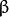一般称作 增益 和 偏置 参数。我们往往用这两个参数来分别控制 对比度 和 亮度 。
你可以把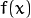看成源图像像素，把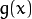看成输出图像像素。这样一来，上面的式子就能写得更清楚些：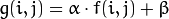其中，和表示像素位于 第i行 和 第j列 。
3、运行代码如下：
程序使用三种方式来实现亮度和对比度的调整，并且输出了各自运算的时间。
#include "stdafx.h"

#include <opencv2/core/core.hpp>
#include <opencv2/highgui/highgui.hpp>
#include <iostream>

using namespace std;
using namespace cv;

double alpha; /**< 控制对比度 */
int beta;  /**< 控制亮度 */

int main( int argc, char** argv )
{
double t;
/// 读入用户提供的图像
Mat image = imread( "Lena.jpg" );
Mat new_image = Mat::zeros( image.size(), image.type() );
Mat new_image1 = Mat::zeros( image.size(), image.type() );
Mat new_image2 = Mat::zeros( image.size(), image.type() );

/// 初始化
cout << " Basic Linear Transforms " << endl;
cout << "-------------------------" << endl;
cout << "* Enter the alpha value [1.0-3.0]: ";
cin >> alpha;
cout << "* Enter the beta value [0-100]: ";
cin >> beta;

t = (double)getTickCount();
/// 执行运算 new_image(i,j) = alpha*image(i,j) + beta
for( int y = 0; y < image.rows; y++ )
{
for( int x = 0; x < image.cols; x++ )
{
for( int c = 0; c < 3; c++ )
{
new_image.at<Vec3b>(y,x)[c] = saturate_cast<uchar>( alpha*( image.at<Vec3b>(y,x)[c] ) + beta );
}
}
}
t = 1000*((double)getTickCount() - t)/getTickFrequency();
cout << ".at+[] Times passed in milliseconds: " << t << endl;

t = (double)getTickCount();
int nr= image.rows; // number of rows
int nc= image.cols * image.channels(); // total number of elements per line
for (int y=0; y<nr; y++)
{
uchar* data= image.ptr<uchar>(y);
uchar* data1=new_image1.ptr<uchar>(y);
for (int x=0; x<nc; x++)
{
data1[x]=saturate_cast<uchar>(alpha*data[x]+beta);
}
}
t = 1000*((double)getTickCount() - t)/getTickFrequency();
cout << ".ptr+[] Times passed in milliseconds: " << t << endl;

t = (double)getTickCount();
image.convertTo(new_image2, -1, alpha, beta);
t = 1000*((double)getTickCount() - t)/getTickFrequency();
cout << "convertTo Times passed in milliseconds: " << t << endl;

/// 创建窗口
namedWindow("Original Image", 1);
namedWindow("New Image", 1);
namedWindow("New Image1", 1);
namedWindow("New Image2", 1);

/// 显示图像
imshow("Original Image", image);
imshow("New Image", new_image);
imshow("New Image1", new_image1);
imshow("New Image2", new_image2);

/// 等待用户按键
waitKey();
return 0;
}


4、运行结果：图1、原图                       图2、.at方法图3、.ptr方法                    图4、convertTo方法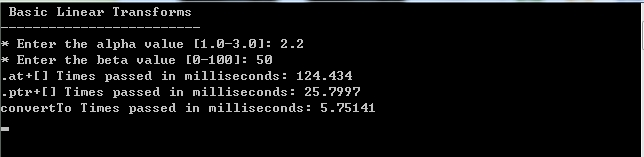图5、运行时间
5、结论
从运行时间可以看出，使用OpenCV自带的函数运行效率最高，而使用.ptr方法比.at方法好，这也印证了前面博客学习OpenCV范例（二）——OpenCV如何扫描图像、利用查找表和计时所说的。
6、用到的类
convertTo：
功能：通过缩放比例将数组变换成其他类型
结构：
void Mat::convertTo(OutputArray m, int rtype, double alpha=1, double beta=0 ) constm：输出图像

rtype：输出图像的类型
alpha：比例因子α，决定对比度
beta：附加值β，决定亮度
函数原理如下：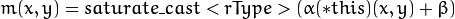saturate_cast：
功能：防止数据溢出
为什么上面的函数会用到saturate_cast呢，因为无论是加是减，乘除，都会超出一个像素灰度值的范围（0～255）所以，所以当运算完之后，结果为负，则转为0，结果超出255，则为255。

展开全文OpenCV convertTo 图像处理
• 图像编辑之对比度调整 亮度对比度的算法公式

继续图片编辑系列的文章，这次讲讲对图片对比度的调整方法。开篇先闲话一番，讲讲一些相关的东西。先是TinyImage的进度，因为某次莫名其妙用另外一个文件把一个很重要的头文件给覆盖了，导致出来一堆编译错误，改了大半个小时，于是为了保险起见赶紧整了个SVN。其次是本来这个周想写写色彩平衡的东西—-上星期主要在研究GIMP中关于色彩平衡实现的代码，但是因为各种原因：周六一大早折腾起来买票，搞得一天都没精神，周日又要去周老师家腐败，没啥心思整理相应的东西，所以先讲讲简单的东西。
对比度，具体的概念解释可以参考Wiki或者百度百科。简单的讲对比度反应了图片上亮区域和暗区域的层次感。而反应到图像编辑上，调整对比度就是在保证平均亮度不变的情况下，扩大或缩小亮的点和暗的点的差异。既然是要保证平均亮度不变，所以对每个点的调整比例必须作用在该值和平均亮度的差值之上，这样才能够保证计算后的平均亮度不变，故有调整公式：

Out = Average + (In – Average) * ( 1 + percent)
其中In表示原始像素点亮度，Average表示整张图片的平均亮度，Out表示调整后的亮度，而percent即调整范围[-1,1]。证明这个公式的正确性相当简单：
设图上有n个像素点，各个点亮度为Ai，平均亮度为A，变化率为alpha，则有：但是实际处理中，并没有太多的必要去计算一张图的平均亮度：一来耗时间，二来在平均亮度上的精确度并不会给图像的处理带来太多的好处—-一般就假设一张图的平均亮度为128，即一半亮度，而一张正常拍照拍出来的图平均亮度应该是在[100,150]。在肉眼看来两者基本没有任何区别，而如果真实地去计算平均亮度还会带来很大的计算量。如下：
通过计算平均亮度来调整对比度

view source

print
?

01void    AdjustContrastUsingAverageThreshold(TiBitmapData& bitmap,doublelevel)

02{ 

03 

04    TINYIMAGE_ASSERT_VOID(level >= -1.0 && level <= 1.0);

05 

06    doublerThresholdSum = 0,gThresholdSum = 0,bThresholdSum = 0;

07    doubledetal= level + 1;

08    intwidth    = bitmap.GetWidth();

09    intheight    = bitmap.GetHeight();

10    intstride    = bitmap.GetStride();

11    intbpp        = bitmap.GetBpp();

12    u8* bmpData    = bitmap.GetBmpData();

13    intoffset    = stride - width * bpp;

14    longpixels = bitmap.GetTotalPixels();

15 

16    for(inti = 0; i < height; i ++) 

17    { 

18        for(intj = 0; j < width; j++) 

19        {

20            rThresholdSum += bmpData[rIndex];

21            gThresholdSum += bmpData[gIndex];

22            bThresholdSum += bmpData[bIndex];

23            bmpData += bpp;

24        }

25        bmpData += offset;

26    } 

27 

28    intrThreshold = (int)(rThresholdSum/pixels);

29    intgThreshold = (int)(gThresholdSum/pixels);

30    intbThreshold = (int)(bThresholdSum/pixels);

31 

32    u8 r_lookup,g_lookup,b_lookup;

33 

34    for(inti = 0; i < 256; i++) 

35    { 

36        r_lookup[i] = (u8)CLAMP0255(rThreshold + (i - rThreshold)* detal);

37        g_lookup[i] = (u8)CLAMP0255(gThreshold + (i - gThreshold)* detal);

38        b_lookup[i] = (u8)CLAMP0255(bThreshold + (i - bThreshold)* detal);

39    } 

40 

41    AdjustCurve(bitmap,r_lookup,g_lookup,b_lookup);

42}

不计算平均亮度：

view source

print
?

01void    AdjustContrastUsingConstThreshold(TiBitmapData& bitmap,doublelevel)

02{ 

03 

04    TINYIMAGE_ASSERT_VOID(level >= -1.0 && level <= 1.0);

05 

06    u8 lookup;

07    doubledelta        = 1 + level;

08    constintthreshold = 0x7F;//128 可以认为是平均亮度

09 

10    for(inti = 0; i < 256; i++) 

11    { 

12        lookup[i] = (u8)CLAMP0255(threshold + (i - threshold)* delta);

13    } 

14 

15    AdjustCurve(bitmap,lookup,TINYIMAGE_CHANEL_RGB);

16}

而在调用算法的时候完全可以通过一个开关来控制到底是调用哪个—-个人推荐下一种，虽然不严格符合调整对比度的语义，但效果基本一致。在Release下下一种基本是瞬间完成，对于3K*2K的图也能保证在100ms内完成。

view source

print
?

1void    AdjustContrast(TiBitmapData& bitmap,doublelevel)

2{ 

3#ifdef CONSTTHRESHOLD 

4    AdjustContrastUsingConstThreshold(bitmap,level);

5#else 

6    AdjustContrastUsingAverageThreshold(bitmap,level);

7#endif 

8}

下面对亮度/对比度的原理简单介绍一下。
一、Photoshop对比度算法。可以用下面的公式来表示：
(1)、nRGB = RGB + (RGB - Threshold) * Contrast / 255
公式中，nRGB表示图像像素新的R、G、B分量，RGB表示图像像素R、G、B分量，Threshold为给定的阀值，Contrast为处理过的对比度增量。
Photoshop对于对比度增量，是按给定值的正负分别处理的：
当增量等于-255时，是图像对比度的下端极限，此时，图像RGB各分量都等于阀值，图像呈全灰色，灰度图上只有1条线，即阀值灰度；
当增量大于-255且小于0时，直接用上面的公式计算图像像素各分量；
当增量等于 255时，是图像对比度的上端极限，实际等于设置图像阀值，图像由最多八种颜色组成，灰度图上最多8条线，即红、黄、绿、青、蓝、紫及黑与白；
当增量大于0且小于255时，则先按下面公式(2)处理增量，然后再按上面公式(1)计算对比度：
(2)、nContrast = 255 * 255 / (255 - Contrast) - 255
公式中的nContrast为处理后的对比度增量，Contrast为给定的对比度增量。
二、图像亮度调整。本文采用的是最常用的非线性亮度调整（Phoposhop CS3以下版本也是这种亮度调整方式，CS3及以上版本也保留了该亮度调整方式的选项），本文亮度调整采用MMX，对亮度增量分正负情况分别进行了处理，每次处理2个像素，速度相当快，比常规BASM代码的亮度处理过程还要快几倍（参见《GDI+ 在Delphi程序的应用 -- 调整图像亮度》）。
三、图像亮度/对比度综合调整算法。这个很简单，当亮度、对比度同时调整时，如果对比度大于0，现调整亮度，再调整对比度；当对比度小于0时，则相反，先调整对比度，再调整亮度。

亮度对比度的算法公式
一副图像的亮度对比度调节属于图像的灰度线性变换，其公式如下:
y = [x - 127.5 * (1 - B)] * k + 127.5 * (1 + B); x为调节前的像素值，y为调节后的像素值。
其中B取值[-1,1]，调节亮度；
k调节对比度，arctan(k)取值[1,89]，所以
k = tan( (45 + 44 * c) / 180 * pi );
其中c取值[-1,1]。通常我们用该值来设置对比度
特别的,
当B=0 时：y = (x - 127.5) * k + 127.5; 这时只调节对比度。
当c=0 时，k = 1：y = x + 255 * B; 这时只调节亮度。

评论这张转发至微博转发至微博

再分享一下我老师大神的人工智能教程吧。零基础！通俗易懂！风趣幽默！还带黄段子！希望你也加入到我们人工智能的队伍中来！https://blog.csdn.net/jiangjunshow
展开全文• f(i,j)源图像像素,g(i,j)目标图像像素，ij表示像素位于第i行第j列，α用来调节图像对比度，β调节图像亮度。 本程序可以通过两个滑动条分别调节图像的对比度亮度。此外，还能分别处理单通道和三通道图像。特别...
今天学完了调整图像对比度与亮度之后，想与大家一起分享一下学习经验。
首先，我们先来介绍下原理吧。主要是利用以下公式：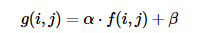f(i,j)源图像像素,g(i,j)目标图像像素，i和j表示像素位于第i行第j列，α用来调节图像对比度，β调节图像亮度。 本程序可以通过两个滑动条分别调节图像的对比度与亮度。此外，还能分别处理单通道和三通道图像。特别说明 cv::Mat::convertTo函数也能实现此功能，且效率比访问像素值要高。（在本程序最后有具体实现，效果和访问像素值的一样） 主要的API： 1、saturate_cast<uchar>(value)确保值大小范围为0~255。 2、Mat.at<Vec3b>(y,x)[index]=value 给每个像素点每个通道赋值 3、void cv::Mat::convertTo  ( OutputArray  m,     int  rtype,     double  alpha = 1,     double  beta = 0    )  const  该方法将源像素值转换为目标数据类型。在末尾应用了saturate_cast<> ，以避免可能的溢出: m(x,y)= saturate_cast < rType >(α(∗)(x,y)+β) 参数
m 输出矩阵;如果在操作前没有适当的大小或类型，则重新分配。
rtype 期望的输出矩阵类型。
alpha 可选的比例因子。 beta 可选的添加到缩放值的增量
实现代码：

#include<opencv2/opencv.hpp>
#include<iostream>
using namespace cv;
using namespace std;
//--------【定义窗口名称】-------------------
char in[] = "source image";
char out[] = "brightness_contrast_improvement";
//---------【声明全局变量】------------------
int alpha = 1;
int bate = 30;
int CONTRAST_MAX = 50;
int BRIGHTNESS_MAX = 150;
Mat srcImage, dstImage,dst_convert;
//---------【声明全局函数】------------------
void ContrastBrightnessImprovement(int,void*);
//----------【主函数】----------------------
int main(int argc, char** argv)
{
//cvtColor(srcImage, srcImage, CV_BGR2GRAY);//将原图转化为灰度图
dstImage = Mat::zeros(srcImage.size(),srcImage.type());
if (!srcImage.data)
{
cout << "could not load image" << endl;
return -1;
}
namedWindow(in, CV_WINDOW_AUTOSIZE);
imshow(in, srcImage);
namedWindow(out, CV_WINDOW_AUTOSIZE);
//创建调整对比度和亮度的滑动条
createTrackbar("contrast", out, &alpha, CONTRAST_MAX, ContrastBrightnessImprovement);
createTrackbar("brightness", out, &bate, BRIGHTNESS_MAX, ContrastBrightnessImprovement);
ContrastBrightnessImprovement(0, 0);
waitKey(0);
return 0;
}
//调整图像亮度和对比度
void ContrastBrightnessImprovement(int, void*)
{
int rows = srcImage.rows;
int cols = srcImage.cols;
for (int row = 0; row < rows; row++) {
for (int col = 0; col < cols; col++)
{
if (srcImage.channels() == 3)  //处理RGB三通道图像
{
int b = srcImage.at<Vec3b>(row, col);
int g = srcImage.at<Vec3b>(row, col);
int r = srcImage.at<Vec3b>(row, col);
dstImage.at<Vec3b>(row, col) = saturate_cast<uchar>(b/10.0*alpha + bate);
dstImage.at<Vec3b>(row, col) = saturate_cast<uchar>(g/10.0*alpha + bate);
dstImage.at<Vec3b>(row, col) = saturate_cast<uchar>(r/10.0*alpha + bate);
}
else if (srcImage.channels() == 1) //处理灰度图
{
int gray = srcImage.at<uchar>(row, col);
dstImage.at<uchar>(row, col) = saturate_cast<uchar>(gray/10.0*alpha + bate);
}
}
}
//使用convertTo函数实现
srcImage.convertTo(dst_convert, -1, alpha/10.0, bate);
imshow("dst_convert", dst_convert);
imshow(out, dstImage);
}

运行结果：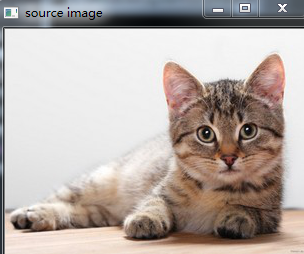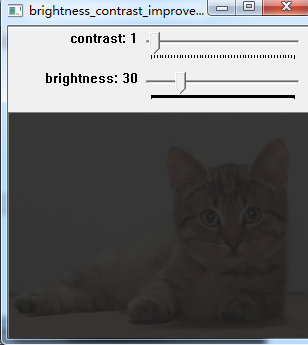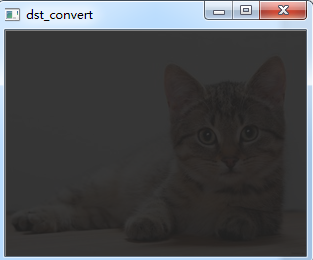展开全文OPenCV3.2
• 基本原理：两张图片合成。  先按照原来的图片的格式新建一个色素全为零的图片，然后按照两张图的比例不同合成一张新图片。...#粗略的调节对比度和亮度 def contrast_brightness_image(src1, a, g): ...
• 基本原理：两张图片合成。  先按照原来的图片的格式新建一个色素全为零的图片，然后按照两张图的比例不同合成一张新图片。...#粗略的调节对比度和亮度 def contrast_brightness_image(src1, a, g)...
• 图像亮度对比度的调整，是放在skimage包的exposure模块里面 1、gamma调整 原理：I=Ig 对原图像的像素，进行幂运算，得到新的像素值。公式中的g就是gamma值。 如果gamma>1, 新图像比原图像暗 如果gamma 函数...
• skimage包的全称是scikit-image SciKit (toolkit...它是由python语言编写的，由scipy 社区开发维护。skimage包由许多的子模块组成，各个子模块提供不同的功能。主要子模块列表如下： 子模块名称　主要实现功能 io ...python 图像强度
• 当要减少对比度的时候，则增加亮度值比中值小的值，降低亮度值比中值更大的值，使所有亮度值向中值靠近。 根据公式（x-avg_R(G/B)*a + x (a 为-1到1 之间，x 是原像素值)计算 亮度的调节： 介绍 将图像转化为HLS格式...饱和度调节 灰度化
• 图像亮度对比度的调整，是放在skimage包的exposure模块里面 一 gamma调整 原理：I=Ig 对原图像的像素，进行幂运算，得到新的像素值。公式中的g就是gamma值。 如果gamma>1, 新图像比原图像暗 如果gamma<...
• 在上篇文章中，我们介绍了如何用filter2D函数来对...下面将以改变图像的对比度和亮度来举例。 改变图像的对比度和亮度，归根到底，就是下面的这个数学公式： 这个式子很好理解。就是将原来图像中的像素值进行比例变换opencv
• 对图片对比度和亮度的调整一般通过下面公式计算： g ( x , y ) = a ∗ f ( x , y ) + b g(x,y) = a*f(x,y)+b g ( x , y ) = a ∗ f ( x , y ) + b f ( x , y ) f(x,y) f ( x , y ) 代表源图像 x行，y列的像素...批量读取文件
• 理解图像中基本概念：色调、色相、饱和度、对比度亮度 对比度对比度指不同颜色之间的差别。对比度越大，不同颜色之间的反差越大，即所谓黑白分明，对比度过大，图像就会显得很刺眼。对比度越小，不同颜色之间...
• 图像亮度对比度的调整，是放在skimage包的exposure模块里面 1、gamma调整 原理：I=Ig 对原图像的像素，进行幂运算，得到新的像素值。公式中的g就是gamma值。 如果gamma>1, 新图像比原图像暗 如...
• 图像变换可以看做两部分： ...调整图像亮度和对比度属于像素变换 g(i,j)=αf(i,j)+βg\left ( i,j \right )=\alpha f\left ( i,j \right )+\beta 其中(i,j)\left ( i,j \right )表示图像上的坐标点，α>0\OpenCV笔记
• 上一篇文章，我们讲解了图像处理中的卷积操作平滑（也就是模糊）处理，这篇文章我们进行亮度和对比度的变化。 其实，亮度是啥玩意？ 亮度就是比较亮眼咯…… 实际上对于RGBA颜色空间，变亮其实就等于R、G、B三个...
• 但如果你的男朋友掌握不好角度，亮度和对比度，那可能就是大型拍照翻车现场了。 你眼中的自己男友眼中的你 好吧，说回正题，如果我们不考虑拍的丑不丑，我们只想让图片亮度对比度调整一下，还是可以做到滴！接...伽马校正
• 亮度是指画面的明亮程度，单位是堪...更精准地说，对比度就是把白色信号在100%0%的饱和度相减，再除以用Lux(光照度，即勒克斯，每平方米的流明值)为计量单位下0%的白色值(0%的白色信号实际上就是黑色)，所得到的...
• 版权声明：转载请注明原作者及出处本文所涉及的一切图像像素运算，前提都是两张图尺寸相同，通道数相同import numpy ...算术运算有加减乘除，加权叠加，可以用来调节亮度和对比度""" """python3 opencv 计算机视觉 图像处理
•图像处理
• 图像亮度对比度的调整，是放在skimage包的exposure模块里面 1、gamma调整 原理：I=Ig 对原图像的像素，进行幂运算，得到新的像素值。公式中的g就是gamma值。 如果gamma&gt;1, 新图像比原图像暗 如果gamma...
• 来自CSDN博客：... 如何通过调节gamma曲线以调节图像的对比度亮度等参数 本文来自CSDN博客，转载请标明出处：http://blog.csdn.net/ipromiseu/archive/2010/10/11/5934064.aspx 作者 Gray Luo(gu...